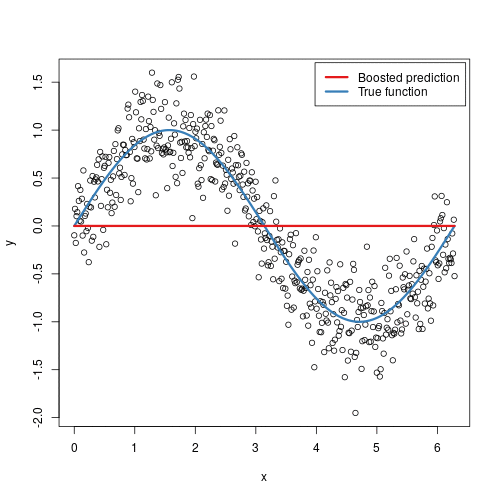# Gradient Boosting Machines

## Gradient Boosting Machines

######Illustration taken from http://uc-r.github.io/gbm_regression

Boosting is a method of converting weak learners into strong learners. In boosting, each new tree is a fit on a modified version of the original data set. The gradient boosting algorithm (gbm) can be most easily explained by first introducing the AdaBoost Algorithm. The AdaBoost Algorithm begins by training a decision tree in which each observation is assigned an equal weight. After evaluating the first tree, we increase the weights of those observations that are difficult to classify and lower the weights for those that are easy to classify. The second tree is therefore grown on this weighted data. Here, the idea is to improve upon the predictions of the first tree. Our new model is therefore Tree 1 + Tree 2. We then compute the classification error from this new 2-tree ensemble model and grow a third tree to predict the revised residuals. We repeat this process for a specified number of iterations. Subsequent trees help us to classify observations that are not well classified by the previous trees.

Gradient Boosting trains many models in a gradual, additive and sequential manner. The major difference between AdaBoost and Gradient Boosting Algorithm is how the two algorithms identify the shortcomings of weak learners (eg. decision trees). While the AdaBoost model identifies the shortcomings by using high weight data points, gradient boosting performs the same by using gradients in the loss function (y=ax+b+e, e needs a special mention as it is the error term). The loss function is a measure indicating how good are model’s coefficients are at fitting the underlying data. So, the basic intuition behind gradient boosting algorithm is to repetitively leverage the patterns in residuals in order to minimize our loss function, such that test loss reach its minima. A logical understanding of loss function would depend on what we are trying to optimize. For example, if we are trying to predict the sales prices by using a regression, then the loss function would be based off the error between true and predicted house prices. One of the biggest motivations of using gradient boosting is that it allows one to optimize on different loss functions and provides several hyper parameter tuning options that make the function fit very flexible

I hope that this blog helped you to get basic intuition behind how gradient boosting works. To understand gradient boosting in detail, I would strongly recommend you to read my complete article: https://www.kdnuggets.com/2019/02/understanding-gradient-boosting-machines.html

Thank You

By: Harshdeep Singh